# Consecutive Numbers That Add up to 684

684 is made from three consecutive even numbers so it is divisible by 3. The number in the middle of those consecutive numbers is divisible by 3 so 684 is also divisible by 9.

684 can be expressed as the sum of consecutive numbers in several ways:

• 227 + 228 + 229 = 684 because one of its factor pairs is 3 x 228.
• 72 + 73 + 74 + 75 + 76 + 77 + 78 + 79 + 80 = 684 because one of its factor pairs is 9 x 76.
• 82 + 83 + 84 + 85 + 86 + 87 + 88 + 89 = 684 because it is greater than 32, is divisible by 4 but not by 8, and 684/8 = 85 1/2.
• 27  + 28 + 29 + 30 + 31 + 32 + 33 + 34 + 35 + 36 + 37 + 38 + 39 + 40 + 41 + 42 + 43 + 44 + 45 = 684 because one of its factor pairs is 19 x 36.
• 17 + 18 + 19 + 20 + 21 + 22 + 23 + 24 + 25 + 26 + 27 + 28 + 29 + 30 + 31 + 32 + 33 + 34 + 35 + 36 + 37 + 38 + 39 + 40 = 684 because it is greater than 288, is divisible by 12 but not by 24, and 684/24 = 28 1/2.

684 is the sum of consecutive prime numbers 337 and 347.

684 is also the sum of the sixteen prime numbers from 13 to 73. Can you list all those prime numbers?

Best of all, the cubes of consecutive numbers 5, 6, and 7 add up to 684.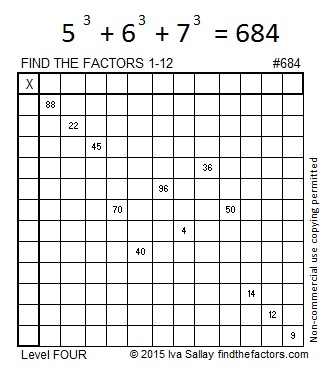Print the puzzles or type the solution on this excel file: 12 Factors 2015-11-16

—————————————————————————————————

• 684 is a composite number.
• Prime factorization: 684 = 2 x 2 x 3 x 3 x 19, which can be written 684 = (2^2) x (3^2) x 19
• The exponents in the prime factorization are 2, 2 and 1. Adding one to each and multiplying we get (2 + 1)(2 + 1)(1 + 1) = 3 x 3 x 2 = 18. Therefore 684 has exactly 18 factors.
• Factors of 684: 1, 2, 3, 4, 6, 9, 12, 18, 19, 36, 38, 57, 76, 114, 171, 228, 342, 684
• Factor pairs: 684 = 1 x 684, 2 x 342, 3 x 228, 4 x 171, 6 x 114, 9 x 76, 12 x 57, 18 x 38 or 19 x 36
• Taking the factor pair with the largest square number factor, we get √684 = (√36)(√19) = 6√19 ≈ 26.15339.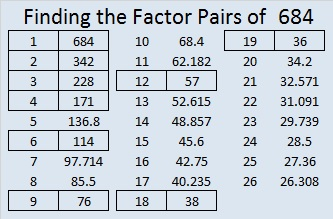—————————————————————————————————# 623 and Level 2

623 is the hypotenuse of Pythagorean triple 273-560-623. What is the greatest common factor of those three numbers?

623 is not divisible by 2, 3, or 5. Is 623 divisible by 7? You can apply either of the following divisibility rules after you separate 623’s digits into 62 and 3:

1st rule: 62 – 2(3) = 62 – 6 = 56. Since 56 is divisible by 7, 623 is divisible by 7.

2nd rule: 62 + 5(3) = 62 + 15 = 77. Since 77 is divisible by 7, 623 is divisible by 7.

86 + 87 + 88 + 89 + 90 + 91 + 92 = 623  (7 consecutive numbers because 623 is divisible by 7)Print the puzzles or type the solution on this excel file: 12 Factors 2015-09-21

—————————————————————————————————

• 623 is a composite number.
• Prime factorization: 623 = 7 x 89
• The exponents in the prime factorization are 1 and 1. Adding one to each and multiplying we get (1 + 1)(1 + 1) = 2 x 2 = 4. Therefore 623 has exactly 4 factors.
• Factors of 623: 1, 7, 89, 623
• Factor pairs: 623 = 1 x 623 or 7 x 89
• 623 has no square factors that allow its square root to be simplified. √623 ≈ 24.9599679.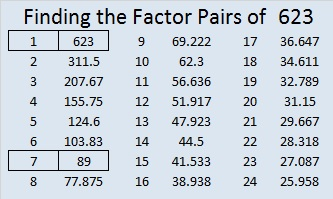—————————————————————————————————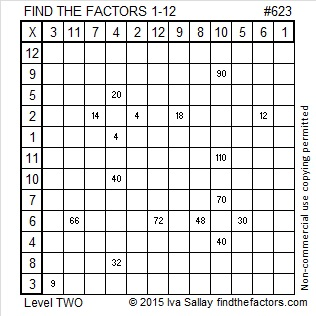# 611 and Level 4

611 is the sum of 13 consecutive numbers:

41 + 42 + 43 + 44 + 45 + 46 + 47 + 48 + 49 + 50 + 51 + 52 + 53 = 611

611 is the sum of 13 consecutive odd numbers:

35 + 37 + 39 + 41 + 43 + 45 + 47 + 49 + 51 + 53 + 55 + 57 + 59 = 611

611 is the sum of the fifteen prime numbers from 13 to 71, a set of numbers that includes all the prime factors of 611.

611 is also the hypotenuse of the Pythagorean triple 235-564-611. What is the greatest common factor of those three numbers?

If you average 13 and 47 (two odd numbers in a factor pair of 611), you get 30 which is 17 numbers away from either factor. That means that (30^2) – (17^2) = 611.

—————————————————————————————————

Today’s puzzle starts off easy enough, but it might get a bit tricky to complete.Print the puzzles or type the solution on this excel file: 12 Factors 2015-09-07

—————————————————————————————————

• 611 is a composite number.
• Prime factorization: 611 = 13 x 47
• The exponents in the prime factorization are 1 and 1. Adding one to each and multiplying we get (1 + 1)(1 + 1) = 2 x 2 = 4. Therefore 611 has exactly 4 factors.
• Factors of 611: 1, 13, 47, 611
• Factor pairs: 611 = 1 x 611 or 13 x 47
• 611 has no square factors that allow its square root to be simplified. √611 ≈ 24.718414.—————————————————————————————————# 597 and Level 5

597 is made from three consecutive odd numbers so it is divisible by 3.

Because it is divisible by 3, it is the sum of three consecutive numbers. It is also the sum of three consecutive odd numbers:

• 198 + 199 + 200 = 597
• 197 + 199 + 201 = 597

Why was 199 the middle number in both of those sums?

Stetson.edu informs us that even though (597!!! + 1) generates an error message on our calculators, it is indeed a very large prime number. Whoever discovered that fact must have been extremely excited.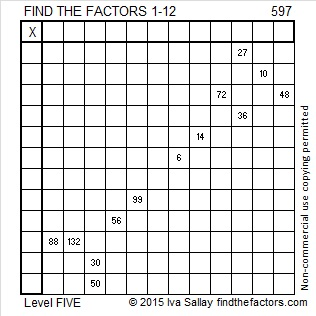Print the puzzles or type the solution on this excel file: 12 Factors 2015-08-24

—————————————————————————————————

• 597 is a composite number.
• Prime factorization: 597 = 3 x 199
• The exponents in the prime factorization are 1 and 1. Adding one to each and multiplying we get (1 + 1)(1 + 1) = 2 x 2 = 4. Therefore 597 has exactly 4 factors.
• Factors of 597: 1, 3, 199, 597
• Factor pairs: 597 = 1 x 597 or 3 x 199
• 597 has no square factors that allow its square root to be simplified. √597 ≈ 24.43358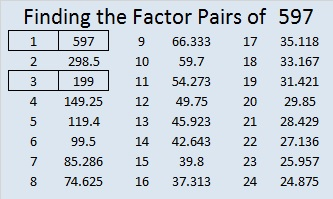—————————————————————————————————# 546 and Level 3

546 is made from 3 consecutive numbers so it is divisible by 3.

The sum of all the prime numbers from 53 to 83 is 546. Can you list all those prime numbers?

546 is the hypotenuse of the Pythagorean triple 210-504-546. Those three numbers have eight common factors, but what is the greatest common factor?

—————————————————————————————————

Puzzles can be a great resource in teaching mathematics. I’ve made crossword puzzles to review vocabulary words before, but none of them looked as inviting to complete as the one Resourceaholic recommended in her 7 July 2015 post on end of term resources. What a difference a little clip art makes to a well-constructed crossword puzzle! The post also includes links to several other amazing mathematics-related puzzles including a polygon word search and a number sequence number search puzzle. Check it out!Print the puzzles or type the solution on this excel file: 10 Factors 2015-07-06

—————————————————————————————————

• 546 is a composite number.
• Prime factorization: 546 = 2 x 3 x 7 x 13
• The exponents in the prime factorization are 1, 1, 1, and 1. Adding one to each and multiplying we get (1 + 1)(1 + 1)(1 + 1)(1 + 1) = 2 x 2 x 2 x 2 = 16. Therefore 546 has exactly 16 factors.
• Factors of 546: 1, 2, 3, 6, 7, 13, 14, 21, 26, 39, 42, 78, 91, 182, 273, 546
• Factor pairs: 546 = 1 x 546, 2 x 273, 3 x 182, 6 x 91, 7 x 78, 13 x 42, 14 x 39, or 21 x 26
• 546 has no square factors that allow its square root to be simplified. √546 ≈ 23.36664—————————————————————————————————

A Logical Approach to solve a FIND THE FACTORS puzzle: Find the column or row with two clues and find their common factor. Write the corresponding factors in the factor column (1st column) and factor row (top row).  Because this is a level three puzzle, you have now written a factor at the top of the factor column. Continue to work from the top of the factor column to the bottom, finding factors and filling in the factor column and the factor row one cell at a time as you go.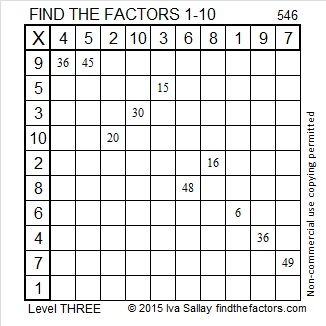# 40% of Numbers Up To 400 Have Square Roots That Can Be Simplified

• 400 is a composite number.
• Prime factorization: 400 = 2 x 2 x 2 x 2 x 5 x 5, which can be written 400 = (2^4) x (5^2)
• The exponents in the prime factorization are 4 and 2. Adding one to each and multiplying we get (4 + 1)(2 + 1) = 5 x 3 = 15. Therefore 400 has exactly 15 factors.
• Factors of 400: 1, 2, 4, 5, 8, 10, 16, 20, 25, 40, 50, 80, 100, 200, 400
• Factor pairs: 400 = 1 x 400, 2 x 200, 4 x 100, 5 x 80, 8 x 50, 10 x 40, 16 x 25, or 20 x 20
• 400 is a perfect square. √400 = 20A few months ago I made a chart showing the number of factors for the first 300 counting numbers. Since this is my 400th post, I’d like to include a chart showing the number of factors for all the numbers from 301 to 400. I’m also interested in consecutive numbers with the same number of factors and whether or not the square root of a number can be reduced. The red numbers have square roots that can be reduced.The longest streak of consecutive numbers with the same number of factors is only three. There are three sets of three consecutive numbers on this chart. (Between 200 and 300 there was a streak of four consecutive numbers with six factors each.)

How do the number of factors of these 100 numbers stack up against the previous 300? The following chart shows the number of integers with a specific number of factors and how many of those integers have reducible square roots:• 39.5% or slightly less than 40% of the numbers up to 400 have reducible square roots.
• Most of these numbers have 2, 4, or 8 factors. Numbers with two factors are prime numbers. Almost all numbers with four factors are the product of two different prime numbers, and nearly two-thirds of the numbers with eight factors are the product of three different prime numbers.
• There isn’t much change between the percentages of reducible square roots from one list to the next.

# 281 and More Consecutive Square Roots

• 281 is a prime number.
• Prime factorization: 281 is prime.
• The exponent of prime number 281 is 1. Adding 1 to that exponent we get (1 + 1) = 2. Therefore 281 has exactly 2 factors.
• Factors of 281: 1, 281
• Factor pairs: 281 = 1 x 281
• 281 has no square factors that allow its square root to be simplified. √281 ≈ 16.763How do we know that 281 is a prime number? If 281 were not a prime number, then it would be divisible by at least one prime number less than or equal to √281 ≈ 16.763. Since 281 cannot be divided evenly by 2, 3, 5, 7, 11, or 13, we know that 281 is a prime number.

So far I have posted about one set of four and two sets of five consecutive reducible square roots. Sets of three consecutive reducible square roots are fairly common so I’ve ignored most of them. These consecutive square roots couldn’t be ignored: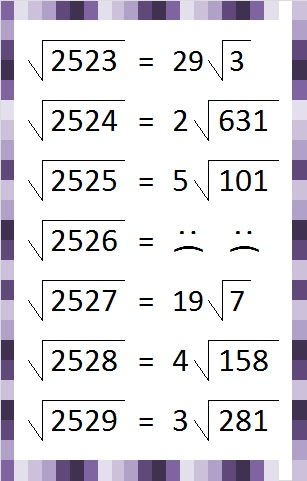The final square root features today’s prime number 281. Here are the prime factorizations and number of factors of each of these numbers:Six is a popular number when counting the number of factors.

# 269 and Five More Consecutive Square Roots

• 269 is a prime number.
• Prime factorization: 269 is prime.
• The exponent of prime number 269 is 1. Adding 1 to that exponent we get (1 + 1) = 2. Therefore 269 has exactly 2 factors.
• Factors of 269: 1, 269
• Factor pairs: 269 = 1 x 269
• 269 has no square factors that allow its square root to be simplified. √269 ≈ 16.401How do we know that 269 is a prime number? If 269 were not a prime number, then it would be divisible by at least one prime number less than or equal to √269 ≈ 16.401. Since 269 cannot be divided evenly by 2, 3, 5, 7, 11, or 13, we know that 269 is a prime number.

As I have previously written, 844, 845, 846, 847, and 848 are the smallest FIVE consecutive numbers whose square roots can be simplified. Here are the second smallest FIVE with the same property.The first number in the second set, 1680, equals 2 x 840 which is very close to the first number in the first set. Will strings of five consecutive numbers with reducible square roots occur about once every 850 numbers?

We can find the number of factors for these numbers by examining their prime factorizations.The number of factors for each of the integers in this second set ranges from 3 to 40. Only two of the integers have the same number of factors. Finding another string of four or more numbers that have reducible square roots as well as the same number of factors may be difficult.

# 266 Why 8 Consecutive Numbers with 6 Factors is Impossible

• 266 is a composite number.
• Prime factorization: 266 = 2 x 7 x 19
• The exponents in the prime factorization are 1, 1, and 1. Adding one to each and multiplying we get (1 + 1)(1 + 1)(1 + 1) = 2 x 2 x 2 = 8. Therefore 266 has 8 factors.
• Factors of 266: 1, 2, 7, 14, 19, 38, 133, 266
• Factor pairs: 265 = 1 x 266, 2 x 133, 7 x 38, or 14 x 19
• 266 has no square factors that allow its square root to be simplified. √266 ≈ 16.3095242, 243, 244, and 245 are the smallest four consecutive numbers that have the same number of factors. Each of them has exactly six factors, and as a result each one of their square roots can be simplified. Is it possible to have a longer string of consecutive numbers with exactly six factors? I don’t know yet. It seems reasonable that it could happen, so I am on the lookout for five, six, or seven consecutive numbers that have exactly six factors.

I won’t bother looking for a string of eight or more numbers with six factors. I already know that would be impossible. Here’s an example illustrating why:846 ruins the run because it has an additional prime factor that doubles the number of factors that 846 has in all. Further down the number line that problem might be overcome in a different set of consecutive numbers.

However, the problem with 848 is a recurring problem that will never be overcome. 848 is divisible by 8, as is every eighth number. The prime factorization of numbers that are divisible by eight must contain a power of two that is greater than or equal to three. Its number of factors calculation would have to be at least (3 + 1)(1 + 1) = 4 x 2 = 8. (The ONLY number divisible by 8 that has exactly 6 factors is 32.)

Even though the numbers from 844 to 848 don’t have the same number of factors, they still have a distinction. They are the smallest five consecutive numbers whose square roots can be simplified!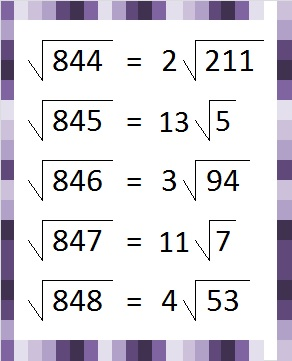# 260 Some Thoughts on Those Four Consecutive Numbers

• 260 is a composite number.
• Prime factorization: 260 = 2 x 2 x 5 x 13, which can be written 260 = (2^2) x 5 x 13
• The exponents in the prime factorization are 2, 1 and 1. Adding one to each and multiplying we get (2 + 1)(1 + 1)(1 + 1) = 3 x 2 x 2 = 12. Therefore 260 has 12 factors.
• Factors of 260: 1, 2, 4, 5, 10, 13, 20, 26, 52, 65, 130, 260
• Factor pairs: 260 = 1 x 260, 2 x 130, 4 x 65, 5 x 52, 10 x 26, or 13 x 20
• Taking the factor pair with the largest square number factor, we get √260 = (√4)(√65) = 2√65 ≈ 16.125Recently I wrote about the smallest four-consecutive-numbers whose square roots could all be simplified. The same numbers were also the smallest four consecutive numbers to have the same number of factors.Each of those numbers had 6 factors, and guess what, ANY number with exactly 6 factors can have its square root simplified. The prime factorization of ANY number with exactly 6 factors can be expressed in one of the three following ways:Since numbers with six factors always have a prime factor raised to a power greater than one, they can always have their square roots simplified. The fact that those four consecutive numbers have the same number of factors makes them extraordinary; that they all can have their square roots simplified is merely the natural consequence of that extraordinary fact.